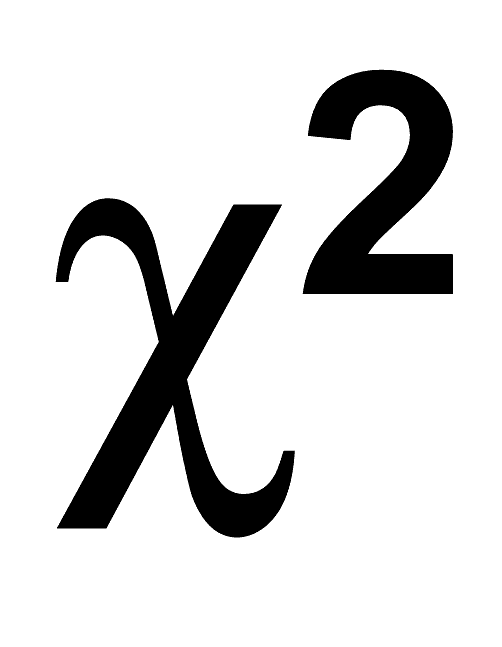Chi-Square Goodness-of-Fit-Test

The chi-square test is a non-parametric test that is used in statistic to determine if an observed distribution or model conforms or is similar to an expected distribution or model. In simple terms, this test will tell you if the data you collected is similar to other data or to what you expected.

There are several types of chi-square test such as the Chi-square Test of Independence, which is used for nominal data, and the Goodness-of-Fit Test,which deals with data that is not nominal. This post is about the Goodness-of-Fit Test. The Goodness-of-Fit test compares the distribution of the observed data with an expected distribution.

A unique caveat of chi-square test is that we normally desire as a researcher to make sure we do not reject our model. This is opposite of traditional hypothesis testing which desires often to reject the null hypothesis as this indicates that there is a statistical difference. With chi-square test, we want our observed model to be similar to the values found in the expected model. What this means is that our model represents what is happening in the real-world and is not only theoretical. If we reject the null it means that the model we are trying to create is not similar to expected values that might be found in the real world. In other words, we found something that does not conform to what is expected. If a model does not represent the world, it may not serve much purpose.

Here are the assumptions of Goodness-of-Fit Test

• Random selection of subjects
• Mutually exclusive categories

Here are the steps

1. Determine hypothesis
• H0: There is no difference between the observed values/model and the expected values/model
• H1: There is a difference between the observed values/model and the expected values/model
2. Decide level of significance
3. Determine degree of freedom to find chi-square critical
4. Compute for the expected frequencies
5. Compute chi-square
6. Make decision to accept or reject null
7. State conclusion

Here is an example

A principal wants to know if the number of students absent each day of the week is the same. Below are the results for one week.

Day                  Absents

Monday                 17

Tuesday                 20

Wednesday            16

Thursday               14

Friday                    13

Step 1: Determine Hypothesis

• H0: The number of students absent is the same every day
• H1: The number of students absent is not the same every day

Step 2: Decide level of significance

• 0.05

Step 3 Determine chi-square critical region (computer does this for you)

• Chi-square critical region = 9.48

Step 4: Compute expected frequencies

• Computer does this

Step 5: Compute Chi square (computer does this for you)

• Chi-square = 1.87

Step 6: Make decision

• Since the computed chi-square of 1,87 is less than the critical chi-square value of 9.48 we do not reject the null hypothesis

Step 7: Conclusion

• Since we do not reject the null hypothesis we can say that there is a lack of evidence that there is a difference in the number of absences each day of the week. In other words, the number of students absent each day is the same.

NOTE: There is also a way to do this test when the expected frequencies are unequal